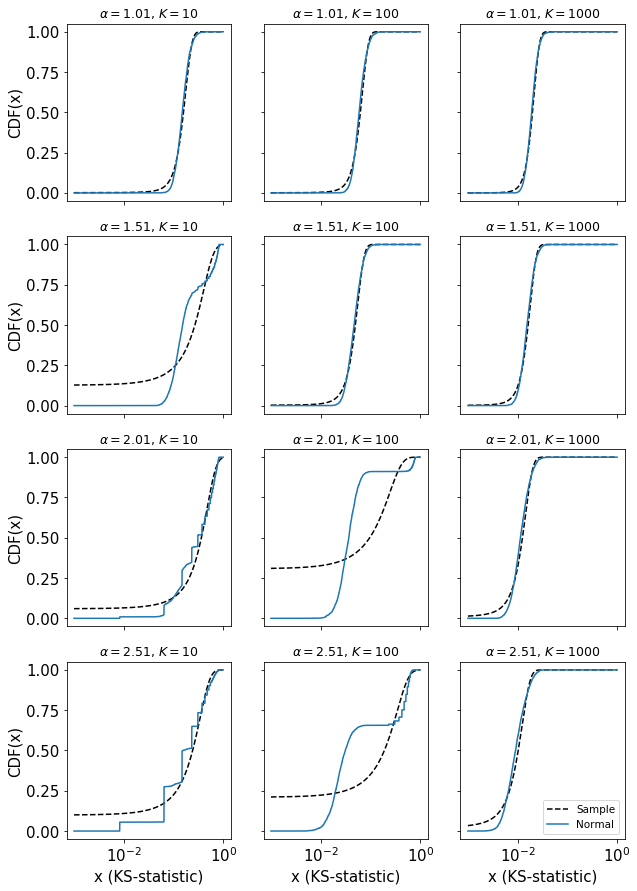# Testing CSN (Clauset, Shalizi, Newman, 2009) method for significance testing.¶

Written by Eddie Lee.

Fitting power laws rigorously is nontrivial. Here's my go at making a pipeline that is easier for myself to use for research using my own implementation. I'm using the misc.stats module that I wrote and available on my GitHub (https://github.com/eltrompetero/misc).

Power laws are of the form
$p(x)\sim x^{-\alpha}$ with $\alpha>1$, lower cutoff $x_0$, and upper cutoff $x_1$. When $x$ is an integer, the normalization can be expressed using a zeta function.

Clauset, A, C R Shalizi, and M E Newman. “Power-Law Distributions in Empirical Data.” Society for Industrial and Applied Mathematics 51.4 (2009): 661–703.

NOTE: If you would like to run this, you will likely need to load a number of modules that I've loaded implicitly.
This is slow! Run parallelized on a fast computer.

In :
%pylab inline

Populating the interactive namespace from numpy and matplotlib

In :
plt.rc('font',size=15)

In :
from misc.stats import DiscretePowerLaw
from statsmodels.distributions import ECDF
import scipy.stats as stats


The significance test involves computing the Kolmogorov-Smirnov (KS) statistic for many random samples where the fitting procedure has been repeated exactly (fitting the lower cutoff and the estimate of the exponent). Importantly, are using the distribution of KS-statistics to determine a p-value, the probability that a random sample happens to be just as similar to the data sample that we have access to.

To get a sense of the KS-statistic across a range of power law exponents and sample sizes, I run the CSN procedure across a range of different values of $\alpha$ and sample sizes $K$.

In :
# specify details of simulation
sampleSizeRange = array([10,100,1000])
alphaRange = arange(1.01, 3, .5)
bootstrapIterations = 2500

# setup
sampleSizeGrid, alphaGrid = meshgrid(sampleSizeRange, alphaRange)
sampleSizeGrid = sampleSizeGrid.ravel()
alphaGrid = alphaGrid.ravel()
ksdistro = zeros((len(alphaGrid), bootstrapIterations))

# run sampling
for i,(s,a) in enumerate(zip(sampleSizeGrid, alphaGrid)):
# obtain distribution of KS statistics for given alpha and sample size
pl = DiscretePowerLaw(alpha=a, lower_bound=1, upper_bound=10_000)
_, ksdistro[i] = pl.clauset_test(ones(s), 0, (1,30), bootstrapIterations)

pickle.dump({'ksdistro':ksdistro,'sampleSizeRange':sampleSizeRange,'alphaRange':alphaRange,
'bootstrapIterations':bootstrapIterations,'sampleSizeGrid':sampleSizeGrid,
'alphaGrid':alphaGrid},
open('csn_powlaw_test.p','wb'), -1)

In :
# using my custom module

Out:
['ksdistro',
'sampleSizeRange',
'alphaRange',
'bootstrapIterations',
'sampleSizeGrid',
'alphaGrid']
In :
# titles specify the exponent value alpha and the sample size K
fig, ax = plt.subplots(figsize=(10,15),
nrows=len(alphaRange), ncols=len(sampleSizeRange), sharex=True, sharey=True)
for i in range(len(ax)):
ax[i,0].set(ylabel='CDF(x)')
for i in range(ax.shape):
ax[-1,i].set(xlabel='x (KS-statistic)')
ax = ax.ravel()

x = logspace(-3,0,10000)  # range of x-axis to show
for i in range(len(ax)):
# show a normal distribution for comparison
ax[i].semilogx(x, stats.norm.cdf(x, scale=ksdistro[i].std(), loc=ksdistro[i].mean()), 'k--')

ecdf = ECDF(ksdistro[i])
ax[i].semilogx(x, ecdf(x))

ax[i].set_title(r'$\alpha=%1.2f$, $K=%d$'%(alphaGrid[i],sampleSizeGrid[i]), fontsize='small')
ax[-1].legend(('Sample','Normal'), fontsize='x-small')

Out:
<matplotlib.legend.Legend at 0x7f1fd2e70e80>In other words, we use the KS-statistic a proxy for how close our sample is to a random sample from a true power law distribution. Picking a significance test using a $p$-value is telling us where is the above CDFs the measured KS-statistic must be to be considered "close enough." Such tests are easier to interpret if the shape of the distribution obeys some intuitive form (e.g. a normal distribution).

Aberrations are more prominent for small sample sizes and larger $\alpha$. Distribution of KS-statistic seems to converge to a nice shape for sample size $K=1000$ and this shown range of $\alpha$. This gives us a sense of the range in which interpreting the KS-statistic is more intuitive although certainly one can use this test even for small samples.

## Some examples¶

A large p-value means that the distribution is statistically indistinguishable from a random sample from a true power law distribution. In other words, p is the probability that a random sample from a power law has a larger KS-statistic.

You can see below that this test seems to work well for a single instance of a power law and an exponential (which is admittedly not very power-law like).

### Power law¶

In :
# solve on a random sample from a power law
X = DiscretePowerLaw.rvs(alpha=2, size=1_000, lower_bound=10, upper_bound=10_000)
alpha, lb = DiscretePowerLaw.max_likelihood(X, upper_bound=10_000, lower_bound_range=(1,30))

# check if it is a reasonable model using Clauset test
pl = DiscretePowerLaw(alpha=alpha,lower_bound=lb, upper_bound=10_000)
ksval = pl.ksval(X)

pval, ksdistro = pl.clauset_test(X[X>=lb], ksval, (1,30), 2500, samples_below_cutoff=X[X<lb])

In :
alpha, lb

Out:
(array([2.1063]), 10)
In :
ksval

Out:
0.019660922447882423
In :
pval

Out:
0.4676

pval is the fraction of random samples that have KS statistic larger than the given sample. When this is close to 1, it means the sample is very similar to a power law distribution. But when it is close to 0, we cannot say with much confidence that the distribution is "close" to a power law. In the paper by CSM, they suggest considering pval>0.1 as likely candidates of power law distributions.

### Power law with lower cutoff¶

Max likelihood correctly identifies the lower bound.

In :
# solve on a random sample from a power law with small number not from distribution
X=concatenate(([1,1,1,2,3,4,5,5,5,5],
DiscretePowerLaw.rvs(alpha=2, size=1_000, lower_bound=10, upper_bound=10_000)))
alpha, lb = DiscretePowerLaw.max_likelihood(X, upper_bound=10_000, lower_bound_range=(1,50))

# check if it is a reasonable model using Clauset test
pl=DiscretePowerLaw(alpha=alpha,lower_bound=lb, upper_bound=10_000)
ksval=pl.ksval(X[X>=lb])

pval, ksdistro = pl.clauset_test(X[X>=lb], ksval, (1,50), 2500, samples_below_cutoff=X[X<lb])

In :
alpha, lb

Out:
(array([2.0269]), 10)
In :
ksval

Out:
0.01731917236467917
In :
pval

Out:
0.6204

### Exponential with lower cutoff¶

As expected, this distribution is strongly different from a power law as reflected in the statistics.

In :
# solve on a random sample from an exponential distribution
X=concatenate(([1,1,1,2,3,4,5,5,5,5],
1+around(random.exponential(scale=100, size=1_000)).astype(int)))
alpha, lb = DiscretePowerLaw.max_likelihood(X, upper_bound=10_000, lower_bound_range=(1,50))

# check if it is a reasonable model using Clauset test
pl=DiscretePowerLaw(alpha=alpha,lower_bound=lb, upper_bound=10_000)
ksval=pl.ksval(X[X>=lb])

pval, ksdistro = pl.clauset_test(X[X>=lb], ksval, (1,50), 2500, samples_below_cutoff=X[X<lb])

In :
alpha, lb

Out:
(array([2.002]), 46)
In :
ksval

Out:
0.15200655683344524
In :
pval

Out:
0.0

### Lognormal¶

In :
# solve on a random sample from a discretized lognormal distribution
X=around(random.lognormal(sigma=3, size=1_000)).astype(int)
X[X==0]=1
alpha, lb = DiscretePowerLaw.max_likelihood(X, upper_bound=inf, lower_bound_range=(1,100))

# check if it is a reasonable model using Clauset test
pl=DiscretePowerLaw(alpha=alpha,lower_bound=lb, upper_bound=inf)
ksval=pl.ksval(X)

pval, ksdistro = pl.clauset_test(X[X>=lb], ksval, (1,100), 250, samples_below_cutoff=X[X<lb])

In :
alpha, lb

Out:
(array([1.5701]), 1)
In :
# ks-statistic seems large because of the max likelihood value found for the lower cutoff lb=1
ksval

Out:
0.12624797869097498
In :
pval

Out:
0.0